# The MCMC Procedure

### Some Useful SAS Functions

Table 61.43: Some Useful SAS Functions

SAS Function

Definition

abs (x);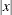airy (x);

Returns the value of the AIRY function.

beta (x1, x2);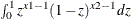call logistic (x);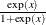call softmax (x1,...,xn);

Each element is replaced by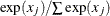call stdize (x1,...,xn);

Standardize values

cdf ();

Cumulative distribution function

cdf (’normal’, x, 0, 1);

Standard normal cumulative distribution function

comb (x1, x2);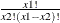constant (’.’);

Calculate commonly used constants

cos (x);

cosine(x)

css (x1, ..., xn);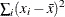cv (x1, ..., xn);

std(x) / mean(x) * 100

dairy (x);

Derivative of the AIRY function

dimN (m);

Returns the numbers of elements in the Nth dim of array m

x1 eq x2

Returns 1 if x1 = x2; 0 otherwise

x1**x2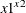geomean (x1, ..., xn);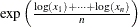difN (x);

Returns differences between the argument and its Nth lag

digamma (x1);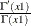erf (x);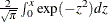erfc (x);

1 - erf(x)

fact (x);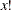floor (x);

Greatest integer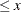gamma (x);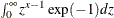harmean (x1, ..., xn);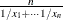ibessel (nu, x, kode);

Modified Bessel function of order nu evaluated at x

jbessel (nu, x);

Bessel function of order nu evaluated at x

lagN (x);

Returns values from a queue

largest (k, x1, ..., xn);

Returns the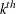largest element

lgamma (x);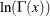lgamma (x+1);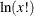log (x, logN(x));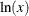logbeta (x1, x2);

lgamma(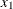) + lgamma(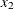) - lgamma(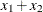)

logcdf ();

Log of a left cumulative distribution function

logpdf ();

Log of a probability density (mass) function

logsdf ();

Log of a survival function

max (x1, x2);

Returnsif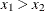;otherwise

mean (of x1-xn);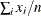median (of x1-xn);

Returns the median of nonmissing values

min (x1, x2);

Returnsif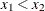;otherwise

missing (x);

Returns 1 if x is missing; 0 otherwise

mod (x1, x2);

Returns the remainder from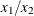n (x1, ..., xn);

Returns number of nonmissing values

nmiss (of y1-yn);

Number of missing values

quantile ();

Computes the quantile from a specific distribution

pdf ();

Probability density (mass) functions

perm (n, r);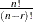put ();

Returns a value that uses a specified format

round (x);

Rounds x

rms (of x1-xn);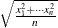sdf ();

Survival function

sign (x);

Returns –1 if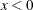; 0 if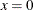; 1 if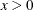sin (x);

sine(x)

smallest (s, x1, ..., en );

Returns the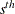smallest component of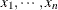sortn (of x1-xn);

Sorts the values of the variables

sqrt (x);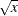std (x1, ..., xn) );

Standard deviation of(n-1 in denominator)

sum (of x:);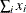trigamma (x);

Derivative of the DIGAMMA(x) function

uss (of x1-xn);

Uncorrected sum of squares

Here are examples of some commonly used transformations:

• logit

mu = beta0 + beta1 * z1;
call logistic(mu);

• log

w = beta0 + beta1 * z1;
mu = exp(w);

• probit

w = beta0 + beta1 * z1;
mu = cdf(normal', w, 0, 1);

• cloglog

w = beta0 + beta1 * z1;
mu = 1 - exp(-exp(w));
`